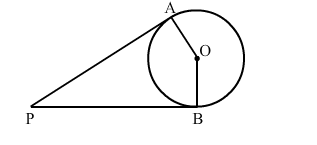# In the given figure, PA and PB are the tangents to a circle with centre O.`
Question:

In the given figure, PA and PB are the tangents to a circle with centre O. Show that the points A, O, B, P are concyclic.Solution:

Here, $O A=O B$

$A$ nd $O A \perp A P, O A \perp B P$ (S ince tangents drawn from an external point are

perpendicular to the radius at the point of contact)

$\therefore \angle O A P=90^{\circ}, \angle O B P=90^{\circ}$

$\therefore \angle O A P+\angle O B P=90^{0}+90^{0}=180^{0}$

$\therefore \angle A O B+\angle A P B=180^{\circ}$ (Since, $\angle O A P+\angle O B P+\angle A O B+\angle A P B=360^{\circ}$ )

Sum of opposite angle of a quadrilateral is $180^{\circ}$.

Hence, $A, O, B$ and $P$ are concyclic.